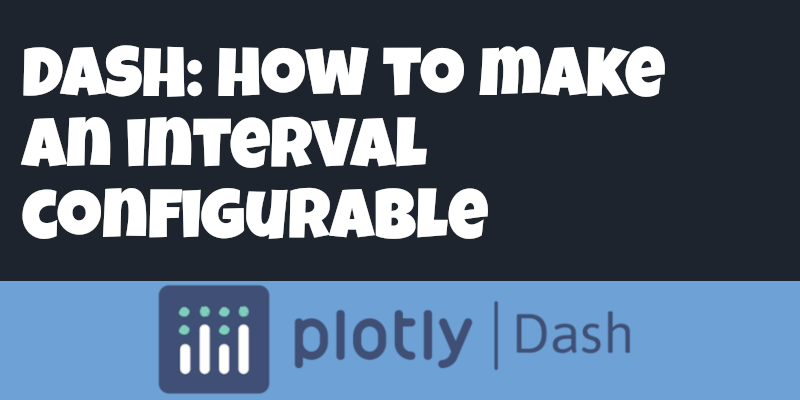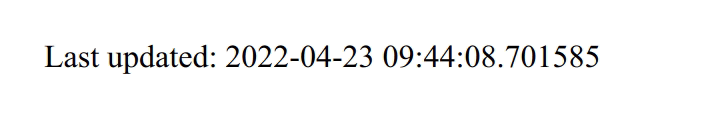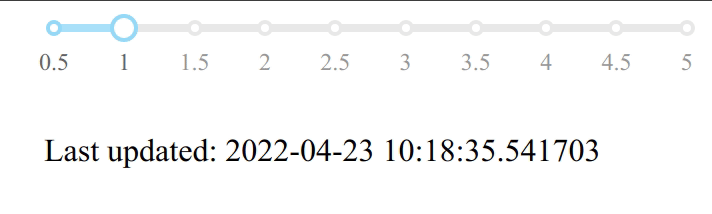# Dash: Configurable dcc.Interval

As I mentioned in my blog post about building a Real-Time Crypto Dashboard, I’ve recently been working with the Dash low-code framework for building interactive data apps.

I was using the `dcc.Interval` component to automatically refresh components on the page and wanted to make the refresh interval configurable. In this blog post we’ll learn how to do that.Figure 1. Dash: Configurable dcc.Interval

## Setup

Let’s first setup our Python environment:

``````python -m venv .env
source .env/bin/activate``````

And install the dash library:

``pip install dash``

## Building a refreshing dashboard

Now we’re going to build a simple dashboard that refreshes a timestamp on the page once every 1,000 milliseconds i.e. once per second. The code to do this is shown below:

dashboard.py
``````import datetime
from dash import Dash, html, dcc, Input, Output

app = Dash(__name__)
app.layout = html.Div([
dcc.Interval(
id='interval-component',
interval=1 * 1000,
n_intervals=0
),
])

@app.callback(
[Output(component_id='latest-timestamp', component_property='children')],
[Input('interval-component', 'n_intervals')]
)
def update_timestamp(interval):
return [html.Span(f"Last updated: {datetime.datetime.now()}")]

if __name__ == '__main__':
app.run_server(debug=True)``````
``python dashboard.py``

If we navigate to http://localhost:8050, we’ll see the timestamp updating once per second, as shown in the following animation:Figure 2. Refreshing every second

The timestamp updates once every second, just as we’d expect.

To make this value configurable we will use `dcc.Slider` to set the new refresh rate and we’ll add a new callback that updates the refresh interval.

dashboard.py
``````import datetime
from dash import Dash, html, dcc, Input, Output

app = Dash(__name__)
app.layout = html.Div([
html.Div(children=[
dcc.Slider(min=0.5, max=5, step=0.5, value=1, id='interval-refresh'), (1)
], style={'width': '20%'}),
dcc.Interval(
id='interval-component',
interval=1 * 1000,
n_intervals=0
),
])

@app.callback(
[Output(component_id='interval-component', component_property='interval')],
[Input('interval-refresh', 'value')])
def update_refresh_rate(value):
return [value * 1000] (2)

@app.callback(
[Output(component_id='latest-timestamp', component_property='children')],
[Input('interval-component', 'n_intervals')]
)
def update_timestamp(interval):
return [html.Span(f"Last updated: {datetime.datetime.now()}")]

if __name__ == '__main__':
app.run_server(debug=True)``````
 1 `dcc.Slider` lets us configure a new refresh rate 2 This callback updates the value of `interval-component`

Now if we navigate back to our web browser, we can adjust the interval to be whatever we like:Figure 3. Refreshing at different intervals